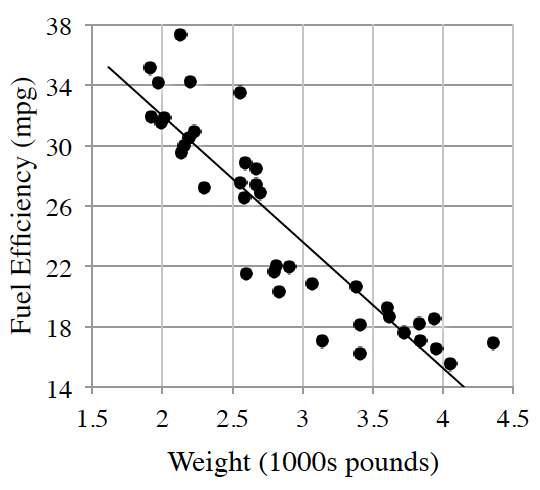Home > CCA > Chapter 4 > Lesson 4.2.3 > Problem4-65

4-65.A consumer magazine collected the following data for the fuel efficiency of cars (miles per gallon) compared to weight (thousands of pounds). Homework Help ✎

• $e=49−8.4w$

• $w$ is the weight ($1000$s of pounds)

• and $e$ is the fuel efficiency (mpg).

1. Describe the association between fuel efficiency and weight.

Moderately strong negative linear association with no apparent outliers.

2. Cheetah Motors has come out with a super lightweight sports utility vehicle (SUV) that weighs only $2800$ pounds. What does the model predict the fuel efficiency will be?

Use the graph to come up with a prediction of the fuel efficiency of the new SUV.